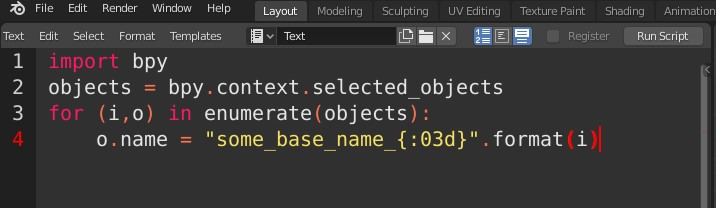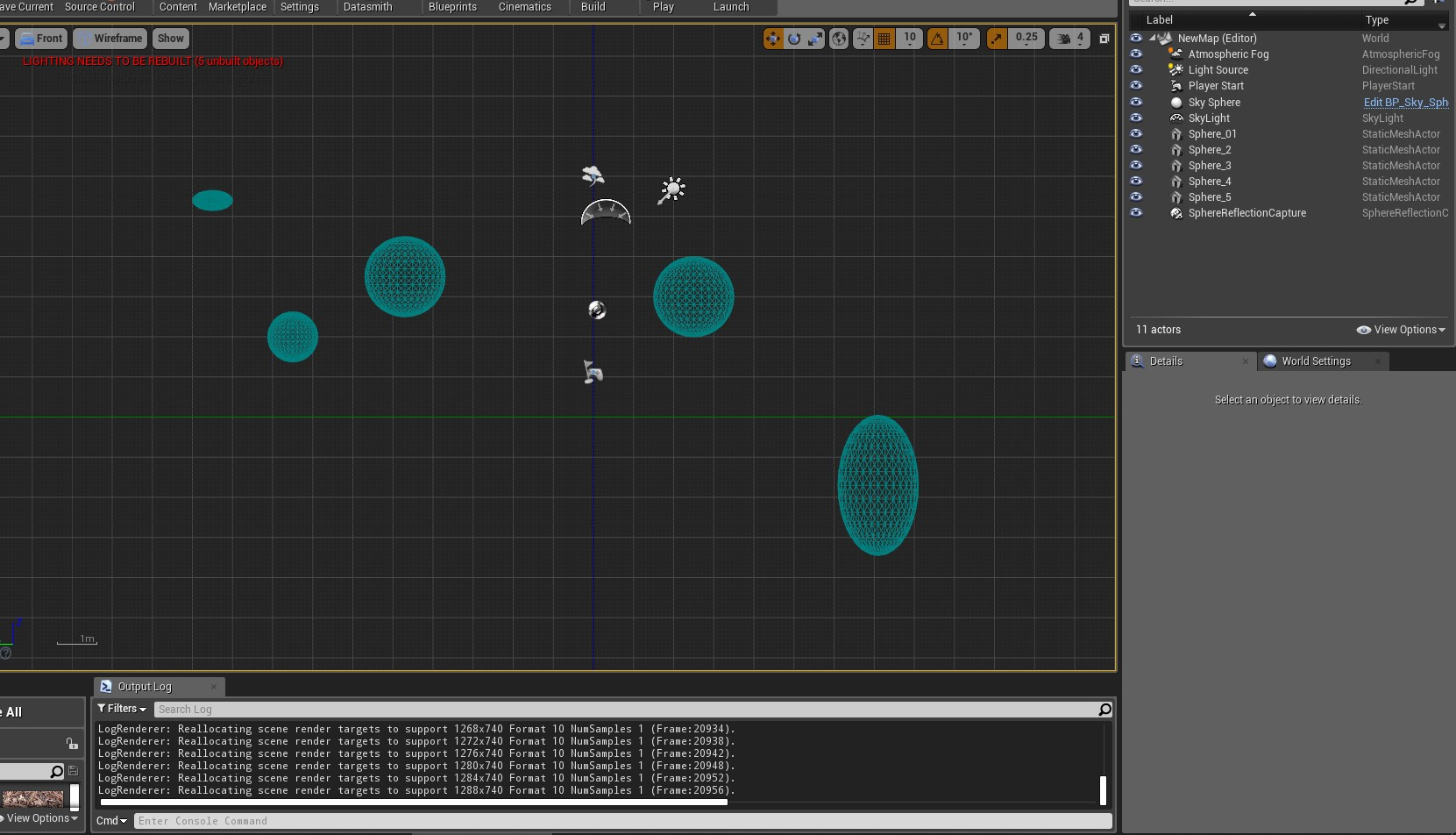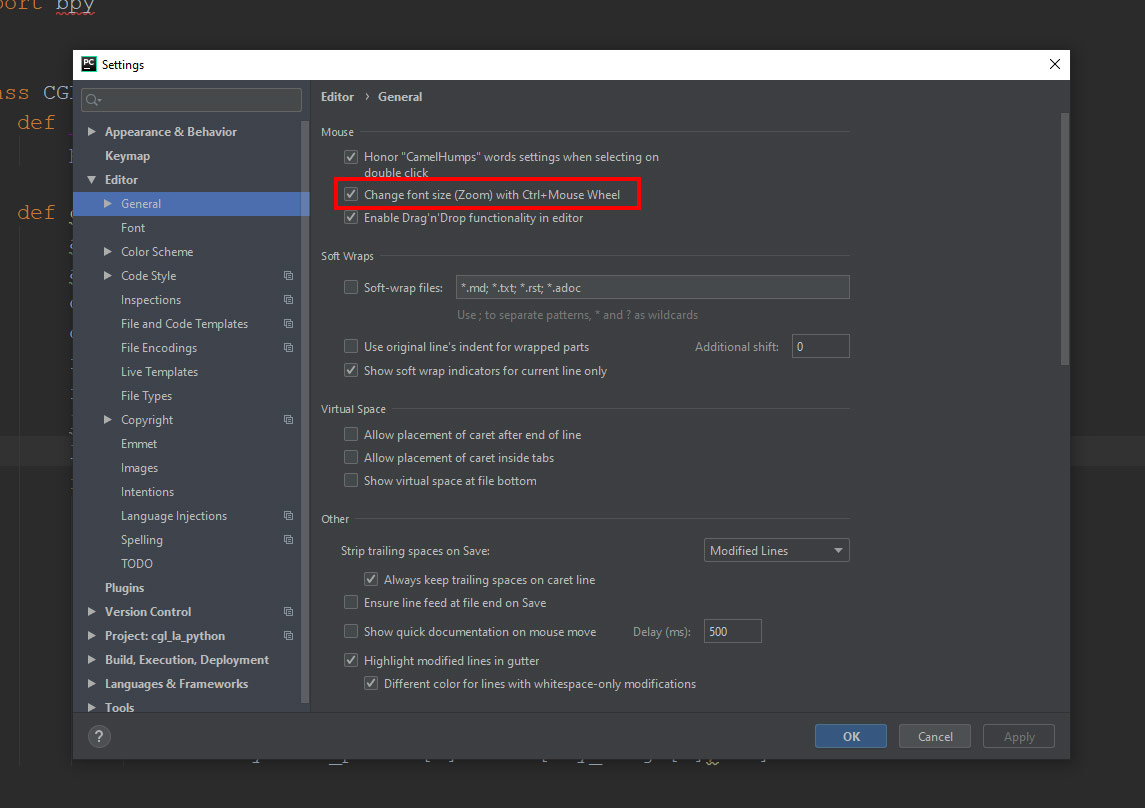## A collection of Python snippets for 3D

If you’re interested in taking the first step into Python for 3D software,
Or simply would like to browse some script examples, your welcome to visit my Gist page,
It contains a useful library of Python code example for Blender, Maya, 3ds max and Unreal engine:
https://gist.github.com/CGLion

## Blender Python – Reading mesh UV data

Software:
Blender 2.83

Simple example code for reading mesh UV data.

Link to this code snippet on gist

Note that there are typically many more mesh loops than vertices.
*Unless the case is a primitive undivided plane..

``````import bpy
access mesh data:
obj = bpy.context.active_object
mesh_data = obj.data
mesh_loops = mesh_data.loops
uv_index = 0
iterate teh mesh loops:
for lp in mesh_loops:
# access uv loop:
uv_loop = mesh_data.uv_layers[uv_index].data[lp.index]
uv_coords = uv_loop.uv
print('vert: {}, U: {}, V: {}'.format(lp.vertex_index,      uv_coords, uv_coords))``````

## Blender – Python – Access animated vertices data

Software:
Blender 2.83

The following is simple example of reading a mesh’s animated vertices data.

This example code gist

Note that accessing an model’s animated vertex locations requires reading the model’s evaluated (deformed) mesh state per frame.
For that reason a new bmesh object is initiated per each frame with the the model’s updated dependency graph.

``````import bpy
import bmesh
obj = bpy.context.active_object
frames = range(0,10)
get the object's evaluated dependency graph:
depgraph = bpy.context.evaluated_depsgraph_get()
iterate animation frames:
for f in frames:
bpy.context.scene.frame_set(f)
# define new bmesh object:
bm = bmesh.new()
bm.verts.ensure_lookup_table()
# read the evaluated mesh data into the bmesh   object:
bm.from_object( obj, depgraph )
# iterate the bmesh verts:
for i, v in enumerate(bm.verts):
print("frame: {}, vert: {}, location: {}".format(f, i, v.co))``````

## Python – Some useful list-of-tuples operations

Language:
Python 3.7

Sometimes we need to sort a list of tuples (or other list-type containers) according to their internal values.
For instance, you might have a list of tuples representing X,Y coordinates and need to sort that list according to the Y coordinate of the locations.
Using the sorted function with a lambda function as the supplied key argument can do that.
In this case, each tuple element is fed to the lambda function as the argument x and the function simply returns x, which is the second (Y) value within the tuple as the key for the sorting comparison:

`sorted(my_tuple_list, key=lambda x: x)`

Again, in a list of tuples (or other list-type containers), Sometimes we need to find the index of the first occurrence of a tuple that has a specific value as one of its elements.
This example will return the index of the first occurrence in the list of a tuple with the value 8 as its second element:

`list(zip(*my_tuple_list)).index(8)`

The zip function “decouples” the tuples into two lists one containing the tuples first element values and the second containing the tuples second element values.
The zip object is fed into a list function to be converted into a subscript-able list containing the 2 new lists, so now we can use the  index to access the list containing only the original tuples’s second elements, and use the index function to get the index of first occurrence of value 8.
> Note that the * operator isn’t a C like “pointer” operator..
Its a Python unpack operator needed to unpack the list of tuples to separate tuple arguments for the zip function.

## Python for 3ds max – Loading an image file and reading pixel values

Software:
3ds max 2020

An example of loading and displaying an image file using Python for 3ds max:
* The EXR image file is located in in the same directory with the 3ds max file in this case.
```from MaxPlus import BitmapManager
image_file_path = r'BG_park_A.exr'
bmp_storage = MaxPlus.Factory.CreateStorage(17)
bmp_info = bmp_storage.GetBitmapInfo()
bmp_info.SetName(image_file_path)
bmp = BitmapManager.Load(bmp_info)
bmp.Display()```

Script explanation line by line:
1. Import the BitmapManager class needed to load image files.
2. Set a variable containing the path to the image file
3. Call the MaxPlus.Factory class’s CreateStorage method to initiate a BitmapStorage object.
This is embarrassing IMO..
And it may very well be that I simply didn’t find the correct way it should be done..
I couldn’t find any other way to independently initiate the BitmapInfo object needed for loading the image, other than Initiating a BitmapStorage object and getting referece to its BitmapInfo object. (the BitmapInfo class has no constructor..)
* If you know a better method to do this I’ll be very grateful if you take the time to comment.
Note:
that the 17 integer argument we supply sets the storage to be compatible with:
32-bit floating-point color depth format (without an alpha channel).
See list of other color format options in this example here:
https://help.autodesk.com/view/3DSMAX/2020/ENU/?guid=__developer_using_maxplus_creating_a_bitmap_html
* They wrote a class containing convenient named constants of the integer arguments (see example code below).
* In this example of creating the BitmapStorage just as a way to generate a BitmapInfo object the actual format you’l supply doesn’t matter, but you can’t use a format that can’t be written to like 8 for example (see list below)
4. Get a reference to the BitmapInfo object contained in the BitmapStorage object.
5. Setting the name property (full file path) of the BitmapInfo object.
6. Loading the image.
7. Displaying the image in 3ds max‘s image viewer window.

Example code for a BitmapStorage format constants container class:

* This example’s source is in the 3ds max help Python examples:
https://help.autodesk.com/view/3DSMAX/2020/ENU/?guid=__developer_using_maxplus_creating_a_bitmap_html
```class BitmapTypes(object):
BMM_NO_TYPE = 0 # Not allocated yet
BMM_LINE_ART = 1 # 1-bit monochrome image
BMM_PALETTED = 2 # 8-bit paletted image. Each pixel value is an index into the color table.
BMM_GRAY_8 = 3 # 8-bit grayscale bitmap.
BMM_GRAY_16 = 4 # 16-bit grayscale bitmap.
BMM_TRUE_16 = 5 # 16-bit true color image.
BMM_TRUE_32 = 6 # 32-bit color: 8 bits each for Red, Green, Blue, and Alpha.
BMM_TRUE_64 = 7 # 64-bit color: 16 bits each for Red, Green, Blue, and Alpha.
BMM_TRUE_24 = 8 # 24-bit color: 8 bits each for Red, Green, and Blue. Cannot be written to.
BMM_TRUE_48 = 9 # 48-bit color: 16 bits each for Red, Green, and Blue. Cannot be written to.
BMM_YUV_422 = 10 # This is the YUV format - CCIR 601. Cannot be written to.
BMM_BMP_4 = 11 # Windows BMP 16-bit color bitmap. Cannot be written to.
BMM_PAD_24 = 12 # Padded 24-bit (in a 32 bit register). Cannot be written to.
BMM_LOGLUV_32 = 13 BMM_LOGLUV_24 = 14
BMM_LOGLUV_24A = 15 BMM_REALPIX_32 = 16 # The 'Real Pixel' format.
BMM_FLOAT_RGBA_32 = 17 # 32-bit floating-point per component (non-compressed),
RGB with or without alpha
BMM_FLOAT_GRAY_32 = 18 # 32-bit floating-point (non-compressed), monochrome/grayscale
BMM_FLOAT_RGB_32 = 19
BMM_FLOAT_A_32 = 20```

Reading pixel values from the image:
```bmp_storage = bmp.GetStorage()
hdr_pixel = bmp_storage.GetHDRPixel(3000,200)
print(hdr_pixel)```
1. Get reference to the Bitmap‘s BitmapStorage object.
* in this case, writing over the BitmapStorage object we created earlier just to get the BitmapInfo object..
2. Read the pixel value.

Note:

When copying and pasting a script from this example, the indentation may not be pasted correctly.

Related:

## Python for Blender – Batch renaming objects

Software:
Blender 2.8 | Python 3.7

Some useful short Python snippets for batch re-naming objects in Blender:

1. Remove last 4 characters from selected object names:
```import bpy
objects = bpy.context.selected_objects
for o in objects:
o.name = o.name[:-4]```2. Rename all selected objects to a set base name followed by a 3 digit numeric suffix:
```import bpy
objects = bpy.context.selected_objects
for (i,o) in enumerate(objects):
o.name = "some_base_name_{:03d}".format(i)```3. Prefix all selected objects name with their Blender data type like renaming “SomeModel” to “MESH_SomeModel” or for example:
```import bpy
objects = bpy.context.selected_objects
for (i,o) in enumerate(objects):
o.name = "{}_{}".format(o.type,o.name)```* note that when copying and pasting a script from this example, the indentation may not be pasted correctly.

Note:
Blender 2.8 has a robust batch renaming utility that is invoked by pressing Ctrl + F2 so we don’t have to write scripts to do batch renaming of objects:## Python for 3ds max – Select objects of type

Software:
3ds max 2020

Continuing this example,
If you need to select objects (nodes) of a certain type i.e. lights, cameras etc.
You can use the INode class’s GetObject() method to get a reference to the node’s object, or “modified object” in 3ds max terms, and use the object’s GetSuperClassID() method to get the integer id representing the object’s superclass.

In addition, the MaxPlus SuperClassIds class contains convenient constants that can be used to avoid having to check and remember the superclasses numeric ids.
See reference here:
https://help.autodesk.com/view/3DSMAX/2017/ENU/?guid=__py_ref_class_max_plus_1_1_super_class_ids_html

An example of a script that selects all light objects in the scene:

```from MaxPlus import SuperClassIds
from MaxPlus import SelectionManager

def scene_objects():
def list_children(node):
list = []
for c in node.Children:
list.append(c)
list = list + list_children(c)
return list
return list_children(MaxPlus.Core.GetRootNode())

for o in scene_objects():
obj = o.GetObject()
if obj.GetSuperClassID() == SuperClassIds.Light:
SelectionManager.SelectNode(o, False)```

* note that when copying and pasting a script from this example, the indentation may not be pasted correctly.

## UE4 – Python – Placing level actors bottom at Z 0.0

Software:
Unreal Engine 4.22

This simple Unreal Editor Python example sets the Z axis location of all actors with names beginning with ‘Sphere_’ in a way that their bottom (minimum Z bound) is at height 0.0.

Download the script

> learn how to run Python scripts in the UE4 Editor

```import unreal
from unreal import Vector

lst_actors = unreal.EditorLevelLibrary.get_all_level_actors()
print('place actors at 0 z')
for act in lst_actors:
act_label = act.get_actor_label()
if 'Sphere_' in act_label:
print('placing: {}'.format(act_label))
act_location = act.get_actor_location()
act_bounds = act.get_actor_bounds(False)
act_min_z = act_bounds.z - act_bounds.z
location_offset = Vector(act_location.x, act_location.y, act_location.z - act_min_z)
act.set_actor_location(location_offset, False, False)```

* note that when copying and pasting a script from this example, the indentation may not be pasted correctly.

Note:
The get_actor_bounds unreal.Actor class method returns a tuple object containing 2 unreal.Vector objects, the first being world space location of the actor geometric center, and the second is the corner of the bounding box relative to the center.

‘Sphere_*’ actors before running the script:‘Sphere_*’ actors after running the script:## Blender Python – Accessing mesh triangles

Software:
Blender 2.81 | Python 3.74

By default, mesh triangles are not accessible to Python in Blender.
When accessing the mesh triangles is needed, they must be calculated first using the ‘calc_loop_triangles’ Mesh object method.
Before the calc_loop_triangle method has been called, the loop_triangles property of the Mesh object will reference a empty collection.
After calling the calc_loop_triangles method, the loop_triangles property will reference a collection of MeshLoopTriangle objects, in which the vertices property will hold an array of 3 integers that are the indices of the triangle vertices.

The following example script creates and places sphere objects at the centers of the cube’s triangles:

```import bpy
mesh = bpy.data.objects['Cube'].data
mesh.calc_loop_triangles()
for tri in mesh.loop_triangles:
tri_center = (mesh.vertices[tri.vertices].co * 0.333) +\
(mesh.vertices[tri.vertices].co * 0.333) +\
(mesh.vertices[tri.vertices].co * 0.333)
bpy.ops.mesh.primitive_uv_sphere_add(radius=0.1,
enter_editmode=False,
location=tri_center)```

* note that when copying and pasting a script from this example, the indentation may not be pasted correctly.

Download and example file here

## PyCharm – Enabling Scroll Zoom

Software:
PyCharm Community 2019.1

Unlike many popular text/programming editors and IDE’s, PyCharm doesn’t by default let you change the font size by pressing Ctrl + Scrolling the mouse wheel.

To enable scroll-zoom in PyCharm:

1. Go to File > Settings to open the Settings dialog
2. In the Settings dialog, navigate to Editor > General
3. Check the Change font size (Zoom) with Ctrl + Mouse Wheel option.